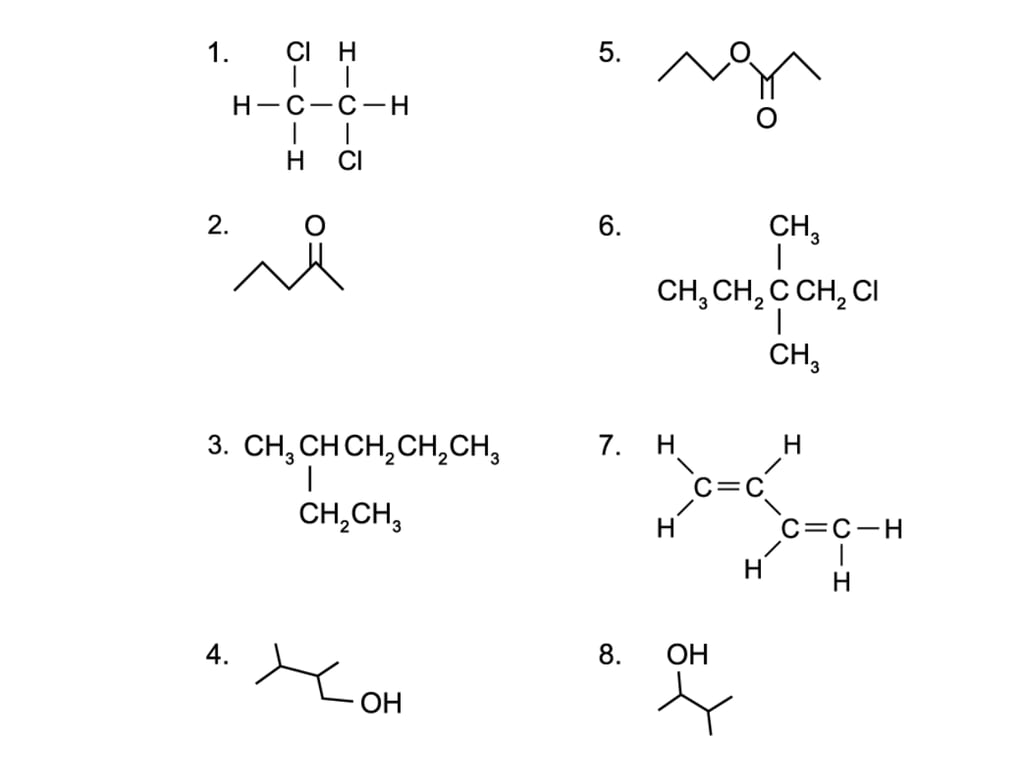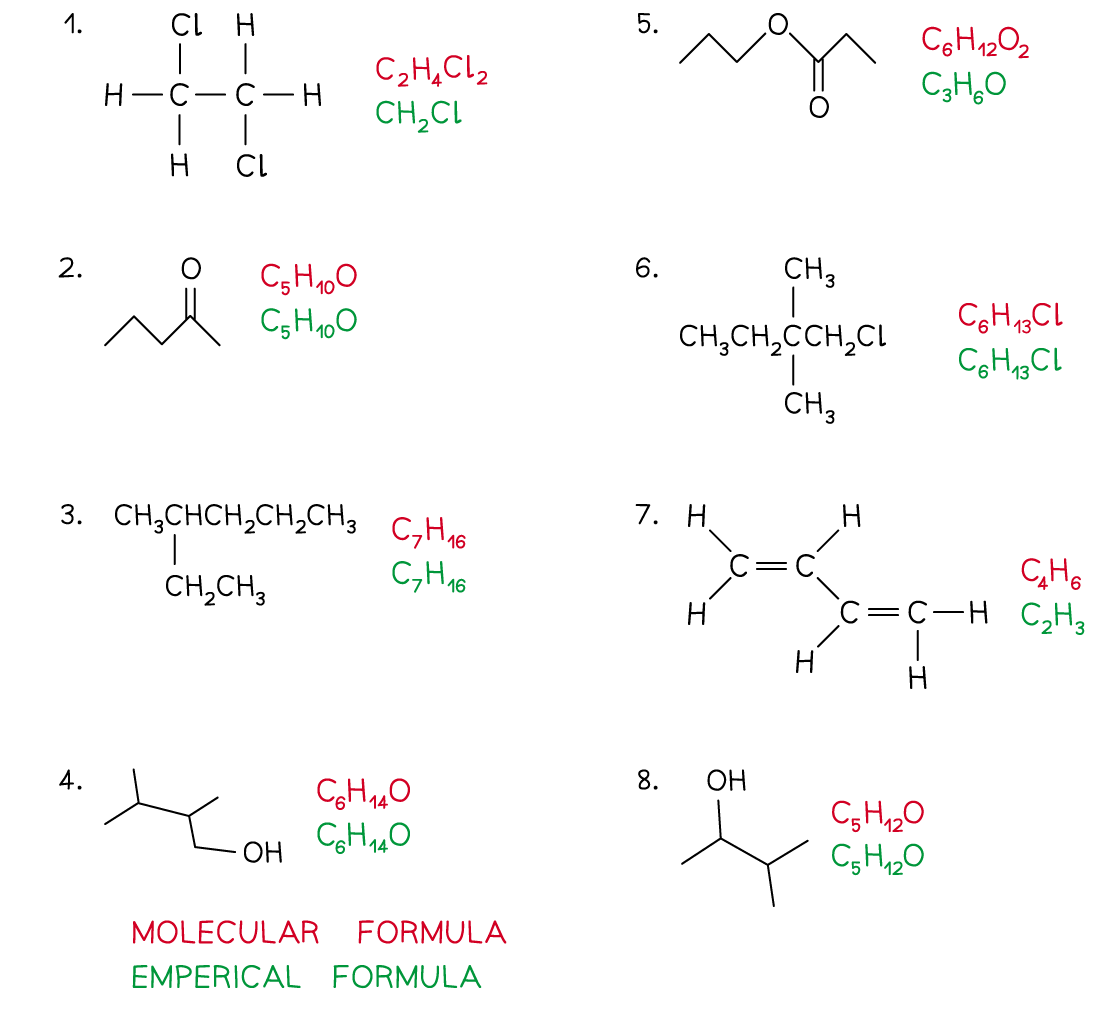# Edexcel A Level Chemistry:复习笔记3.1.1 Formulae of Organic Compounds

### Formulae of Organic Compounds

• Organic chemistry is the chemistry of carbon compounds
• Carbon forms a vast number of compounds because it can form strong covalent bonds with itself
• This enables it to form long chains of carbon atoms, and hence an almost infinite variety of carbon compounds are known
• Hydrocarbons are compounds that are made up of carbon and hydrogen atoms ONLY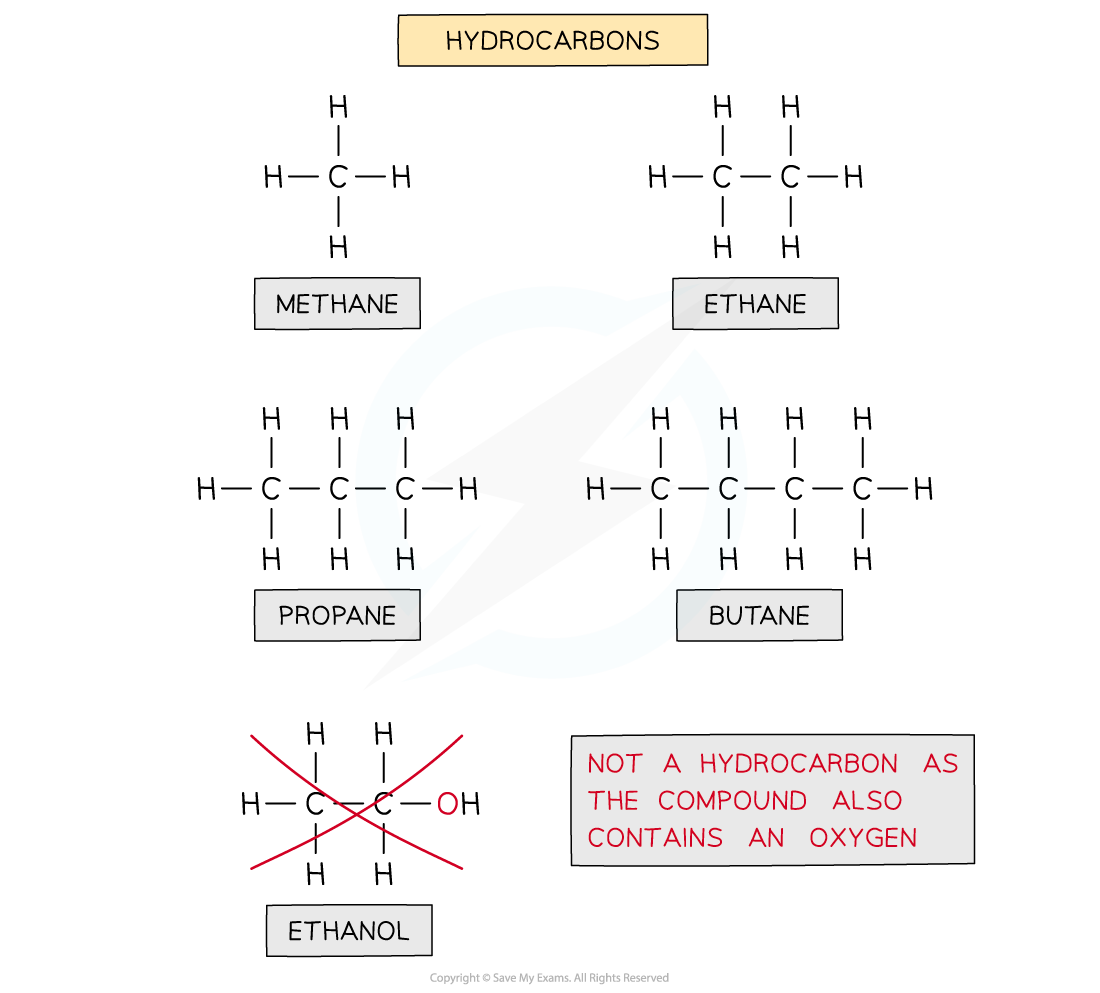The diagram shows examples of hydrocarbons

Ethanol is NOT a hydrocarbon as the molecule also contains an oxygen atom and is not solely made up of carbon and hydrogen

• Hydrocarbons can be placed into two categories
• Saturated molecules containing single carbon-carbon bonds only
• Unsaturated molecules containing double or triple carbon-carbon bonds
• The general formula is a formula that represents a homologous series of compounds using letters and numbers
• Eg. the general formula of alkanes is CnH2n+2
• A homologous series is a group of organic compounds that have the same functional group, the same general formula and the same chemical properties

• The structural formula is a formula that shows how the atoms are bonded to each carbon atom in a molecule
• The displayed formula is a 2D representation of an organic molecule showing all its atoms (by their symbols) and their bonds (by single, double or triple bonds)
• The skeletal formula is a simplified displayed formula with all the carbon and hydrogen (C-H) bonds removed

Overview of the Formulae of Organic Compounds Table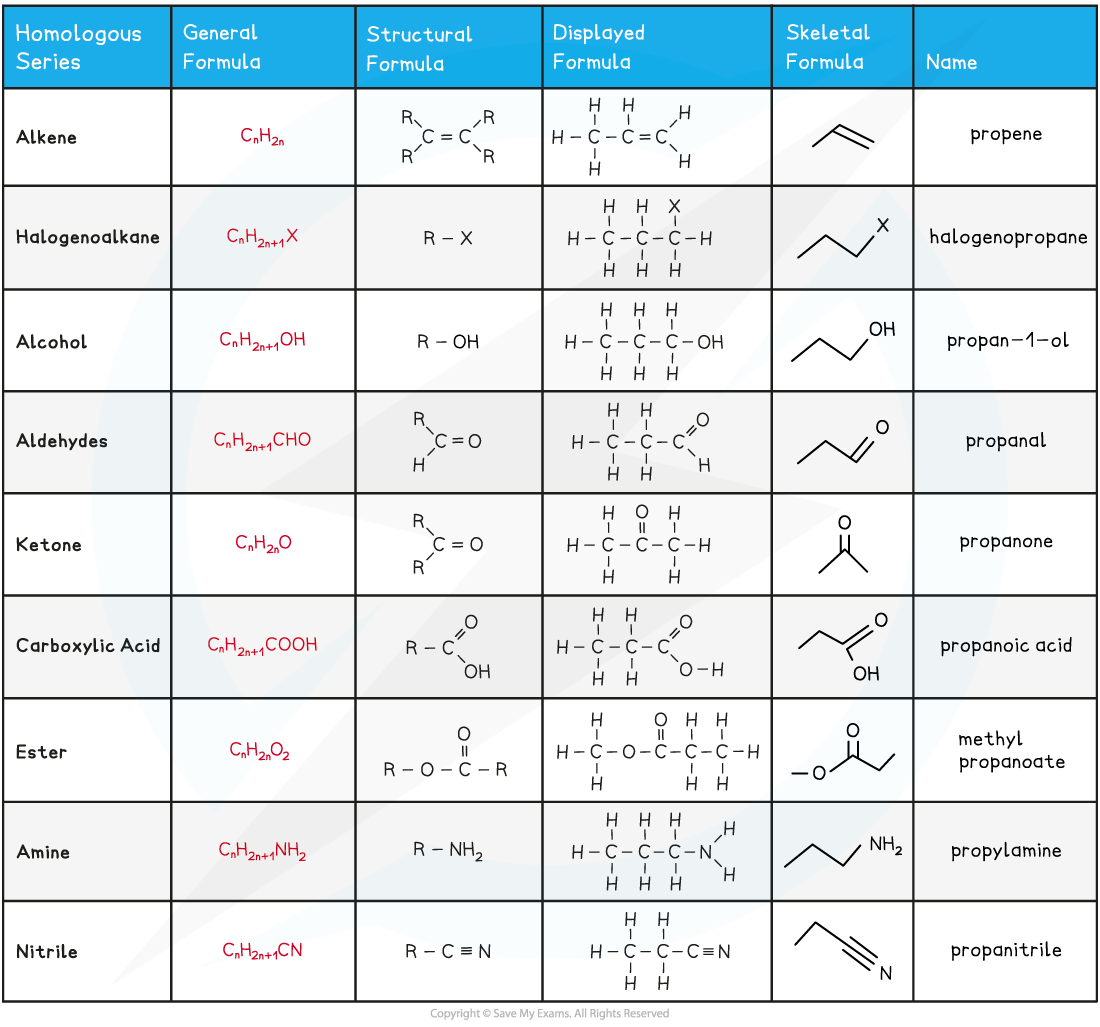#### Worked Example

Drawing skeletal formulae of molecules

Draw the skeletal formula of the following molecules:

1. CH3(CH2)3OH
2. (CH3)2CHCH2OH
3. CH3CH2OCH2CH3#### Worked Example

Drawing displayed formulae of molecules
Draw the displayed formula of the following molecules: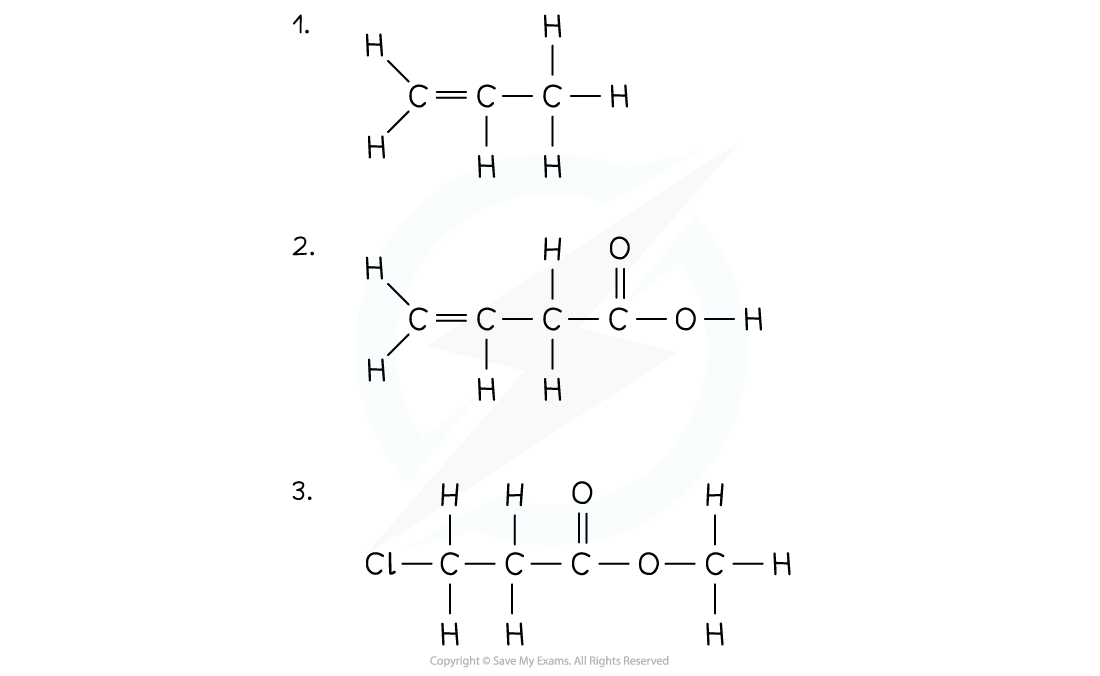• The molecular formula shows the number and type of each atom in a molecule
• Eg. the molecular formula of ethanoic acid is C2H4O2
• The empirical formula shows the simplest whole number ratio of the elements present in one molecule of the compound
• Eg. the empirical formula of ethanoic acid is CH2O

#### Worked Example

Deducing molecular & empirical formulae

Deduce the molecular and empirical formula of the following compounds: